# 5.1: Metal Complexes

$$\newcommand{\vecs}{\overset { \rightharpoonup} {\mathbf{#1}} }$$ $$\newcommand{\vecd}{\overset{-\!-\!\rightharpoonup}{\vphantom{a}\smash {#1}}}$$$$\newcommand{\id}{\mathrm{id}}$$ $$\newcommand{\Span}{\mathrm{span}}$$ $$\newcommand{\kernel}{\mathrm{null}\,}$$ $$\newcommand{\range}{\mathrm{range}\,}$$ $$\newcommand{\RealPart}{\mathrm{Re}}$$ $$\newcommand{\ImaginaryPart}{\mathrm{Im}}$$ $$\newcommand{\Argument}{\mathrm{Arg}}$$ $$\newcommand{\norm}{\| #1 \|}$$ $$\newcommand{\inner}{\langle #1, #2 \rangle}$$ $$\newcommand{\Span}{\mathrm{span}}$$ $$\newcommand{\id}{\mathrm{id}}$$ $$\newcommand{\Span}{\mathrm{span}}$$ $$\newcommand{\kernel}{\mathrm{null}\,}$$ $$\newcommand{\range}{\mathrm{range}\,}$$ $$\newcommand{\RealPart}{\mathrm{Re}}$$ $$\newcommand{\ImaginaryPart}{\mathrm{Im}}$$ $$\newcommand{\Argument}{\mathrm{Arg}}$$ $$\newcommand{\norm}{\| #1 \|}$$ $$\newcommand{\inner}{\langle #1, #2 \rangle}$$ $$\newcommand{\Span}{\mathrm{span}}$$$$\newcommand{\AA}{\unicode[.8,0]{x212B}}$$

Learning Objectives
• To introduce complex ions and the basic principles of metal-ligand bonding

A complex ion has a metal ion at its center with a number of other molecules or ions surrounding it. These can be considered to be attached to the central ion by coordinate (dative covalent) bonds (in some cases, the bonding is actually more complicated than that. The molecules or ions surrounding the central metal ion are called ligands. Simple ligands include water, ammonia and chloride ions.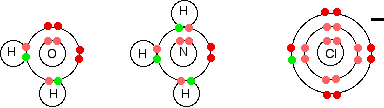Figure $$\PageIndex{1}$$: Simple Bohr diagram bonding in water, ammonia, and the chloride ion.

What all these have got in common is active lone pairs of electrons in the outer energy level. These are used to form co-ordinate bonds with the metal ion. All ligands are lone pair donors. In other words, all ligands function as Lewis bases.

## Bonding in Simple Complex Ions

We are going to look in detail at the bonding in the complex ion formed when water molecules attach themselves to an aluminum ion to give Al(H2O)63+. Start by thinking about the structure of a naked aluminum ion before the water molecules bond to it.

Example $$\PageIndex{1}$$: $$Al(H_2O)_6^{3+}$$

Aluminum has the electronic structure

1s22s22p63s23px1

When it forms an Al3+ ion it loses the 3-level electrons to leave

1s22s22p6

That means that all the 3-level orbitals are now empty. The aluminium uses all six of these empty 3-level orbitals to accept lone pairs from six water molecules. It re-organizes (hybridizes) the 3s, the three 3p, and two of the 3d orbitals to produce six new orbitals all with the same energy.

You might wonder why it chooses to use six orbitals rather than four or eight or whatever. Six is the maximum number of water molecules that will fit around an aluminum ion (and most other metal ions) due to steric constraints. By making the maximum number of bonds, it releases most energy and so becomes most energetically stable.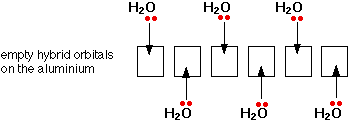Only one lone pair is shown on each water molecule. The other lone pair is pointing away from the aluminum and so isn't involved in the bonding. The resulting ion looks like this: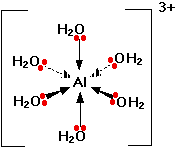Because of the movement of electrons towards the center of the ion, the 3+ charge is no longer located entirely on the aluminum, but is now spread over the whole of the ion. Because the aluminum is forming 6 bonds, the co-ordination number of the aluminum is said to be 6. The co-ordination number of a complex ion counts the number of co-ordinate bonds being formed by the metal ion at its center.

In a simple case like this, that obviously also counts the number of ligands - but that is not necessarily so, as you will see later. Some ligands can form more than one co-ordinate bond with the metal ion.

Example $$\PageIndex{2}$$: $$Fe(H_2O)_6^{3+}$$

Iron has the electronic structure

1s22s22p63s23p63d64s2

When it forms an Fe3+ ion it loses the 4s electrons and one of the 3d electrons to leave

1s22s22p63s23p63d5

Looking at this as electrons-in-boxes, at the bonding level: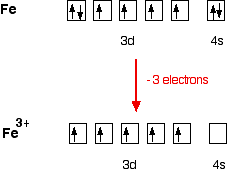The single electrons in the 3d level are NOT involved in the bonding in any way. Instead, the ion uses 6 orbitals from the 4s, 4p and 4d levels to accept lone pairs from the water molecules. Before they are used, the orbitals are re-organized (hybridized) to produce 6 orbitals of equal energy.Once the co-ordinate bonds have been formed, the ion looks exactly the same as the equivalent aluminium ion.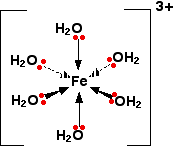Because the iron is forming 6 bonds, the co-ordination number of the iron is 6.

Example $$\PageIndex{3}$$: $$CuCl_4^{2-}$$

This is a simple example of the formation of a complex ion with a negative charge.

Copper has the electronic structure

1s22s22p63s23p63d104s1

When it forms a Cu2+ ion it loses the 4s electron and one of the 3d electrons to leave

1s22s22p63s23p63d9

To bond the four chloride ions as ligands, the empty 4s and 4p orbitals are used (in a hybridized form) to accept a lone pair of electrons from each chloride ion. Because chloride ions are bigger than water molecules, you can't fit 6 of them around the central ion - that's why you only use 4.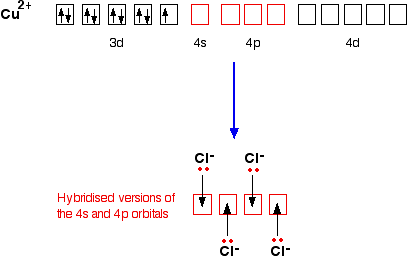Only one of the 4 lone pairs on each chloride ion is shown. The other three are pointing away from the copper ion, and aren't involved in the bonding. That gives you the complex ion:The ion carries 2 negative charges overall. That comes from a combination of the 2 positive charges on the copper ion and the 4 negative charges from the 4 chloride ions. In this case, the co-ordination number of the copper is 4.

## Contributors

5.1: Metal Complexes is shared under a CC BY-NC-SA 4.0 license and was authored, remixed, and/or curated by LibreTexts.